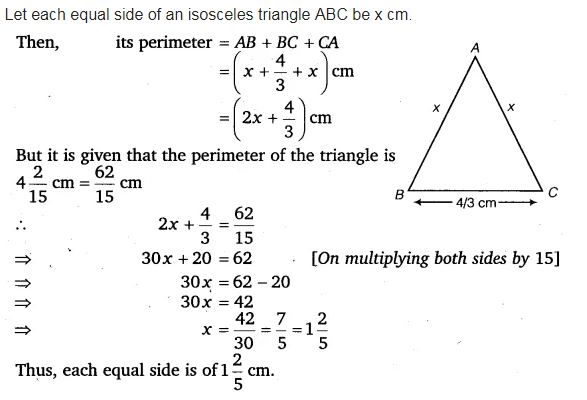# The base of an isosceles triangle is 4/3 cm. The perimeter of the triangle is

The base of an isosceles triangle is 4/3cm. The perimeter of the triangle is 4 2/15cm. What is the length of either of the remaining equal sides ?